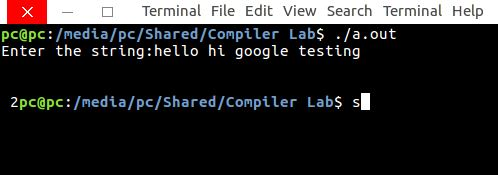# Lex program to count words that are less than 10 and greater than 5

• Last Updated : 10 May, 2019

Problem: Write a Lex program to count words that are less than 10 and greater than 5.

Explanation:
Lex is a computer program that generates lexical analyzers. Lex reads an input stream specifying the lexical analyzer and outputs source code implementing the lexer in the C programming language.

Attention reader! Don’t stop learning now. Get hold of all the important CS Theory concepts for SDE interviews with the CS Theory Course at a student-friendly price and become industry ready.

The commands for executing the lex program are:

```lex abc.l (abc is the file name)
cc lex.yy.c -efl
./a.out
```

Let’s see Lex Program to count words that are less than 10 and greater than 5.

Examples:

```Input: geeksforgeeks hey google test lays
Output: 1 ```

Below is the implementation:

 `/*lex code to count words that are less than 10``    ``- and greater than 5 */`` ` `%{``  ``int` `len=0, counter=0;``%}`` ` `%%``[a-zA-Z]+ { len=``strlen``(yytext);``            ``if``(len<10 && len>5)``              ``{counter++;} }``%%`` ` `int` `yywrap (``void` `)`` ``{``    ``return` `1;`` ``}`` ` `int` `main()`` ``{``  ``printf``(``"Enter the string:"``);``  ``yylex();``  ``printf``(``"\n %d"``, counter);``  ``return` `0;`` ``}`

Output:My Personal Notes arrow_drop_up